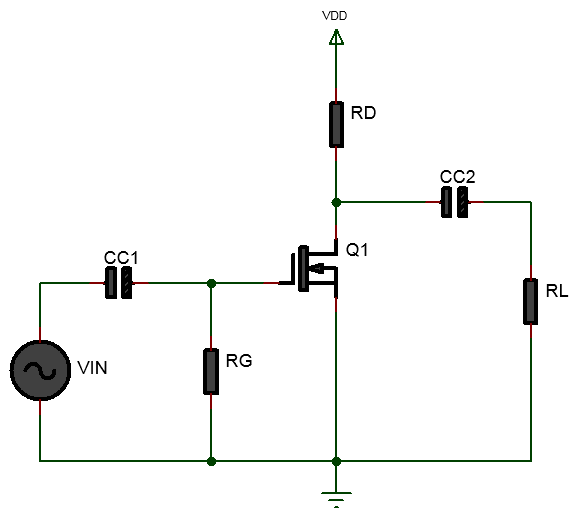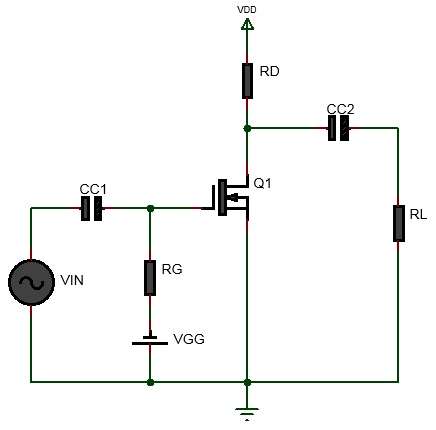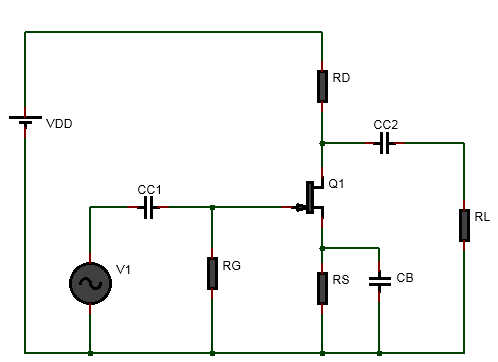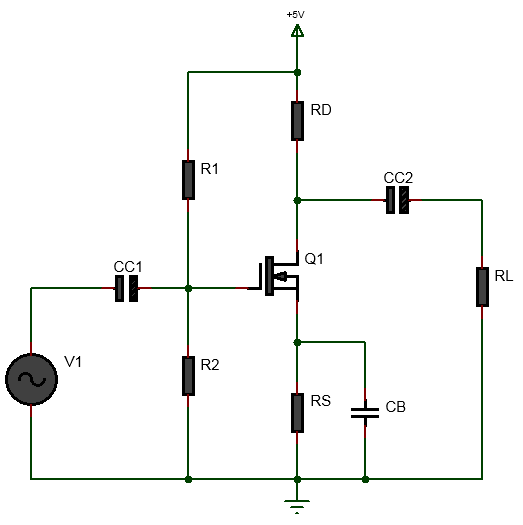# Depletion MOSFET Biasing & Amplifier Design Calculator

## Zero Gate Biased D-MOSFET Calculator

Inputs:

V
mA
V
V

Theoritical Results:### Zero Gate Biased Depletion MOSFET Calculator

The above Zero Gate Biased Depletion MOSFET Calculator helps to calculate the drain current $$I_D$$, , input and output impedance and the coupling capacitors value from $$I_{DSS}$$, the gate voltage which is equal to zero, that is $$V_{G}=0$$ and drain to source voltage $$V_{DS}$$ which is equal to drain voltage $$V_D$$. In zero gate biasing method, a zero gate voltage is applied to the gate and the drain current is equal to the gate shorted drain current value $$I_{DSS}$$. The biasing works even if gate voltage is zero because a depletion MOSFET(D-MOS) can operate with gate to souce junction reversed biased(like JFET) and when the gate to source junction is forward biased. When the gate to souce voltage is revered biased, the depletion MOSFET operates in depletion mode and when the gate to souce voltage is forward biased then the depletion MOSFET works in enhancement mode. Between reverse and forward bias of the gate to souce voltage, the gate is zero and in this situation, the depletion MOSFET still works.
The tutorial Zero Gate Biased Depletion MOSFET Amplifier Design Example explains in details how this calculator was used to calculate the output values.

### Equations Used:

(1) $$I_D = I_{DSS}$$ $$\newline$$ (2) $$R_D=\frac{V_{DD}-V_D}{I_D} \space[assume \space V_D]$$ $$\newline$$ (3) $$r_d = R_D||R_L = \frac{R_D R_L}{R_D+R_L}$$ $$\newline$$ (4) $$g_m = \frac{2 I_{DSS}}{|V_{GS(off)}|}=\frac{2 I_{DSS}}{V_p}$$ $$\newline$$ (5) $$A_v = g_m r_d$$ $$\newline$$ (6) $$Z_i = R_G$$ $$\newline$$ (7) $$Z_o = r_d$$ $$\newline$$ (8)$$CC_1 = \frac{10}{2\pi f Z_i}$$ $$\newline$$ (9) $$CC_2 = \frac{10}{2\pi f Z_o}$$

## Depletion MOSFET Fixed Gate Bias Calculator

Inputs:

V
mA
V
mA
V

Theoritical Results:### Fixed Gate Biased Depletion MOSFET Amplifier & Biasing Calculator

The above depletion MOSFET fixed gate bias calculator helps to calculate the gate to source voltage($$V_{GS}$$) by providing the drain current($$I_D$$), gate shorted drain current($$I_{DSS}$$) and the gate to source cutoff voltage($$V_{GS(off)}$$). By providing the drain voltage($$V_D$$) which is equal to the drain to source voltage( ($$V_{DS}$$)), we can then calculate the drain resistor($$R_D$$). In fixed gate bias, a negative gate to source voltage is applied to the gate to reverse bias the gate to source junction of the depletion MOSFET. When the gate to source junction is reversed biased the depletion MOSFET operates in depletion mode(as opposed to enchancement mode when positive voltage is applied to the gate).The fixed gate bias method is usually used to bias the depletion MOSFET in its ohmic region. It is not suitable for biasing the MOSFET in its active region due to variation of the MOSFET parameters. In ohmic region the MOSFET acts a resistor and a MOSFET biased with gate bias can be used as variable resistor controlled by the gate to source voltage. The tutorial How to bias Depletion MOSFET with fixed gate biasing method explains step by step the process of biasing a depletion MOSFET with gate bias(fixed bias).

### Equations Used:

First obtain $$I_{DSS}$$ and $$V_{GS(off)}$$ from the MOSFET datasheet. $$\newline$$ (1) $$V_{GS} = V_{GS(off)}(1 - \sqrt{\frac{I_D}{I_{DSS}}})$$ $$\newline$$ (2) $$R_D=\frac{V_{DD}-V_{DS}}{I_D}$$ $$\newline$$ (3) $$Z_i = R_G$$ $$\newline$$ (4) $$Z_o = R_D$$ $$\newline$$ (5) $$CC_1 = \frac{10}{2\pi f Z_i}$$ $$\newline$$ (6) $$CC_2 = \frac{10}{2\pi f Z_o}$$

## Depletion MOSFET Self Bias Calculator

Inputs:

V
V
mA
V

Theoritical Results:### Self Biased Depletion MOSFET Amplifier Design Calculator

This self biased Depletion MOSFET amplifier design calculator helps you to bias a Depletion MOSFET using self biasing method. That is it helps to calculate the gate to source voltage to self bias the MOSFET, to calculate the drain current, to calculate the drain to source voltage, the source voltage, helps to calcuate the drain resistor value and the source resistor values, helps to calculate the transconductance. Furthermore it helps to calculate the input and output impedances and the coupling capacitors and bypass capacitor values. A detailed worked out example is shown in the tutorial self biased JFET amplifier design.

### Equations Used:

$$\newline$$ (1) $$V_{GS}=\frac{V_{GS(off)}}{3.4}$$$$\newline$$ (2) $$I_D = \frac{I_{DSS}}{2}$$ $$\newline$$ (3) $$R_S = \frac{V_{GS}}{I_D}$$ $$\newline$$ (4) $$R_D=\frac{V_{DD}-V_{D}}{I_{D}}$$,$$\newline$$ (5) $$g_m = \frac{2 I_{DSS}}{V_p} (1-\frac{V_{GS}}{V_p})$$ $$\newline$$ (6) $$R_d = R_D||R_L = \frac{R_DR_L}{R_D+R_L}$$ $$\newline$$ (7) $$A_v = -g_m R_d$$ $$\newline$$ (8) $$Z_i = R_G \space [as \space R_{GS} \space is \space very \space large]$$ $$\newline$$ (9) $$Z_o = R_D \space [Z_o=r_d||R_D\space assuming \space r_d >> R_D]$$ $$\newline$$ (10) $$CC_1 = \frac{10}{2\pi f Z_i}$$ $$\newline$$ (11) $$CC_2 = \frac{10}{2\pi f Z_o}$$ $$\newline$$

## Voltage Divider Biased Depletion MOSFET Amplifier Calculator

Inputs:

V
V
mA
V

Theoritical Results:### Voltage divider biased Depletion MOSFET amplifier

The Voltage divider biased Depletion MOSFET amplifier calculator above helps to calculate the biasing resistor values and coupling and by-pass capacitor values for given input specification. This is helpful in quick determination for components values for designing Voltage divider biased Depletion MOSFET amplifier. Voltage divider biasing is best suited for stable operation among the biasing methods. For detailed steps and explanations see Voltage Divider Biased D-MOSFET Amplifier Design

### Equations Used:

$$\newline$$ (1) $$V_{GS} = \frac{V_{GS(off)}}{2}$$ $$\newline$$ (2) $$V_{G} = -V_{GS(off)}$$ $$\newline$$ (3) $$I_D=\frac{I_{DSS}}{4}$$ $$\newline$$ (4) $$R_{1}=R_2(\frac{V_{DD}}{V_{G}}-1) \space [assume \space R_2]$$ $$\newline$$ (5) $$V_{S}=\frac{V_G-V_{GS}}{I_D}$$ $$\newline$$ (6) $$R_S = \frac{V_S}{I_D}$$ $$\newline$$ (7) $$R_D = \frac{V_{DD}-V_D}{I_D} \space [assume \space V_D]$$ $$\newline$$ (8) $$r_d = R_D || R_L = \frac{R_D R_L}{R_D+R_L}$$ $$\newline$$ (9) $$g_m = \frac{2 I_{DSS}}{V_p} (1-\frac{V_{GS}}{V_p})$$ $$\newline$$ (10) $$A_v = g_m r_d$$ $$\newline$$ (11) $$Z_i = R_1 || R_2 || R_{GS} \approxeq R_1||R_2 \space [as \space R_{GS} \space is \space very \space large]$$ $$\newline$$ (12) $$Z_o = R_D \space \space[Z_o = r_d||R_D \space assuming \space r_d >> R_D]$$ $$\newline$$ (13) $$CC_1 = \frac{10}{2\pi f Z_i}$$ $$\newline$$ (14) $$CC_2 = \frac{10}{2\pi f Z_o}$$ $$\newline$$ (15) $$CB = \frac{10}{2\pi f R_S}$$ $$\newline$$